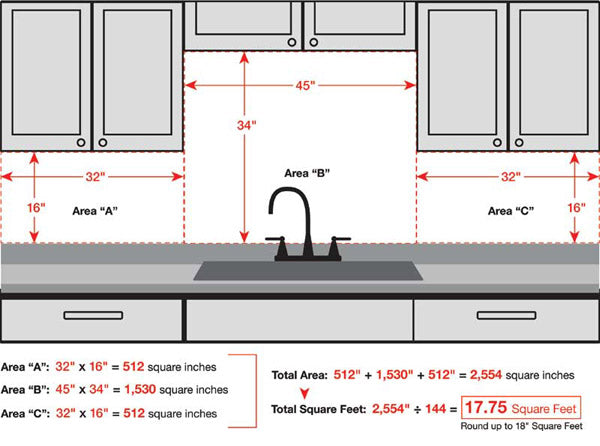# How To Calculate How Much Metal Tile I Need?

How Much Metal Tile Do I Need To Cover My Backsplash Area?

Measuring the area for your new metal backsplash is not complicated. You simply need a measuring tape and a little bit of know-how. We recommend breaking your area up into "rectangles" that you can measure individually. Its easiest to measure in inches, then convert to square feet at the end.

To do this start by measuring the width and height of each area, multiplying the width and height together to get a measurement in square inches.

To convert this measurement to square feet you simply need to divide by 144.

For example, if you have two separate areas, one is 36" wide and 18" tall, and the second is 30" wide and 40" tall. To get the first area in square inches you would simply multiply 36*18 = 648 square inches. The second area would be 30*40 = 1200 square inches.

Together both these areas add up to 1848 square inches (648+1200).

To convert this measurement in square inches to square feet, simply divide by 144, 1848/144 = 12.83 square feet.

In this example, you would need 12.83 square feet of tile, and its best to round up to 13 or 14 square feet to account for overages and errors.

You can then simply order that quantity of metal mosaic tile from this site.

To simplify these instructions, we have provided a diagram and calculation of a sample kitchen backsplash as an example: